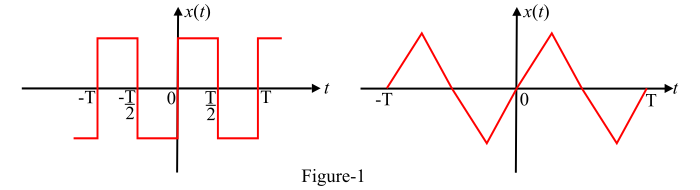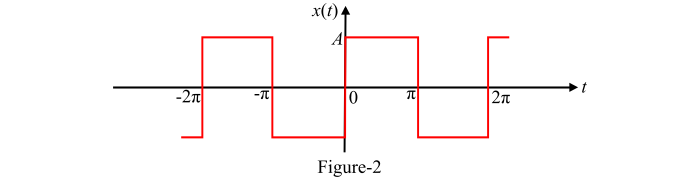# Signals and Systems â€“ What is Quarter Wave Symmetry?

## Quarter Wave Symmetry

A periodic function $x(t)$ which has either odd symmetry or even symmetry along with the half wave symmetry is said to have quarter wave symmetry.

Mathematically, a periodic function $x(t)$ is said to have quarter wave symmetry, if it satisfies the following condition −

$$\mathrm{x(t)=x(-t)\:or\:x(t)=-x(-t)\:and\:x(t)=-x\left (t ± \frac{T}{2}\right )}$$

Some examples of periodic functions having quarter wave symmetry are shown in Figure-1.The Fourier series coefficients for the function having quarter wave symmetry are evaluated as follows −

## Case I – When n is odd

$$\mathrm{x(t)=-x(-t)\:and\:x(t)=-x\left (t ± \frac{T}{2}\right )}$$

For this case,

$$\mathrm{a_{0}=0\:\:and\:\:a_{n}=0}$$

And,

$$\mathrm{b_{n}=\frac{8}{T} \int_{0}^{T/4}x(t)\:sin\:n\omega_{0}\:t\:dt}$$

## Case II – When n is even

$$\mathrm{x(t)=x(-t)\:and\:x(t)=-x\left (t ± \frac{T}{2}\right )}$$

For this case,

$$\mathrm{a_{0}=0\:\:and\:\:b_{n}=0}$$

And,

$$\mathrm{a_{n}=\frac{8}{T} \int_{0}^{T/4}x(t)\:cos\:n\omega_{0}\:t\:dt}$$

## Numerical Example

Find the trigonometric Fourier series for the waveform shown in Figure-2 using the quarter wave symmetry.Solution

From the waveform shown in Figure-2, it is clear that it has odd symmetry along with half wave symmetry because it satisfies the following conditions −

$$\mathrm{x(t)=-x(-t)\:and\:x(t)=-x\left (t ± \frac{T}{2}\right )}$$

Therefore, the given waveform has quarter wave symmetry. Hence, the trigonometric Fourier series coefficients are,

$$\mathrm{a_{0}=0\:\:and\:\:a_{n}=0}$$

And the coefficient $b_{n}$ is given by,

$$\mathrm{b_{n}=\frac{8}{T} \int_{0}^{T/4}x(t)\:sin\:n\omega_{0}\:t\:dt}$$

Also, for this function only odd harmonics exist. Therefore,

• Time period of waveform,

$$\mathrm{T= 2\pi}$$

• Fundamental frequency,

$$\mathrm{\omega_{0}=\frac{2\pi}{T}=\frac{2\pi}{2\pi}=1}$$

$$\mathrm{\therefore\:b_{n}=\frac{8}{T} \int_{0}^{T/4}x(t)\:sin\:n\omega_{0}\:t\:dt=\frac{8}{2\pi}\int_{0}^{\pi/2}A\:sin\:nt\:dt}$$

$$\mathrm{\Rightarrow\:b_{n}=\frac{4A}{\pi}\left [ \frac{-cos\:nt}{n} \right ]_{0}^{\pi/2}=-\frac{4A}{n\pi}[cos(n\pi/2)-cos\:0]}$$

$$\mathrm{\therefore\:b_{n}=\begin{cases}\frac{4A}{n\pi} & for\:odd\:n\0 & for\:even\:n\end{cases}}$$

Therefore, the trigonometric Fourier series for the waveform is,

$$\mathrm{x(t)=\frac{4A}{\pi}sin\:t+\frac{4A}{3\pi}sin\:3t+\frac{4A}{5\pi}sin\:5t+....}$$